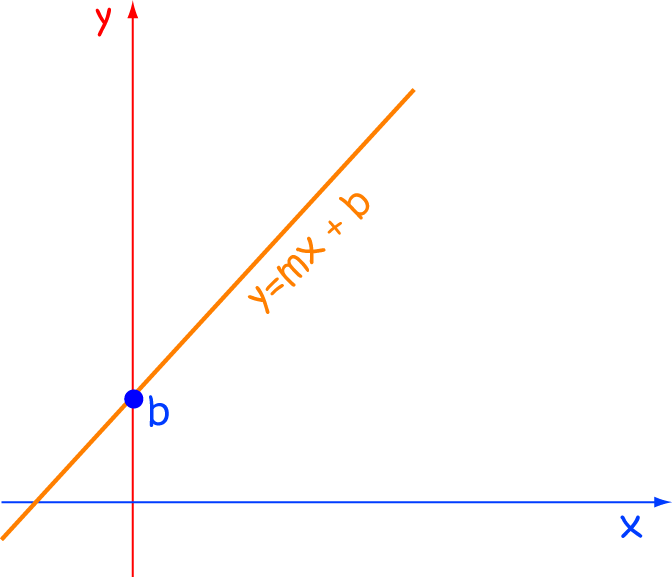# Definition of Linear EquationA linear equation is the equation of a straight line.

It is often written as $y = mx + b$, where $m$ is the slope of the line, and $b$ is the $y$-intercept of the line.

### Description

The aim of this dictionary is to provide definitions to common mathematical terms. Students learn a new math skill every week at school, sometimes just before they start a new skill, if they want to look at what a specific term means, this is where this dictionary will become handy and a go-to guide for a student

### Audience

Year 1 to Year 12 students

### Learning Objectives

Learn common math terms starting with letter L

Author: Subject Coach
You must be logged in as Student to ask a Question.